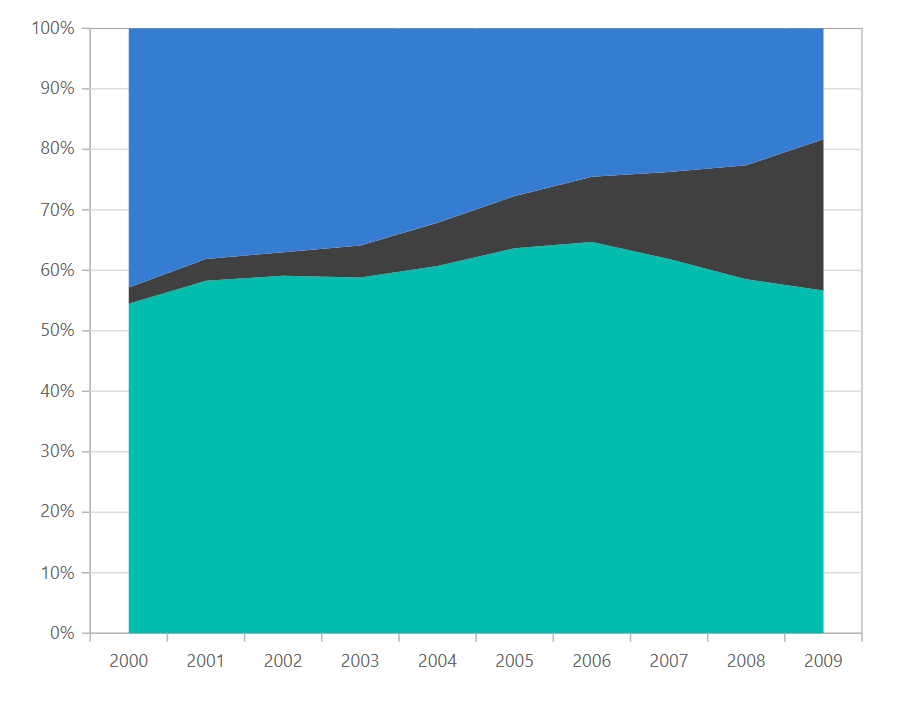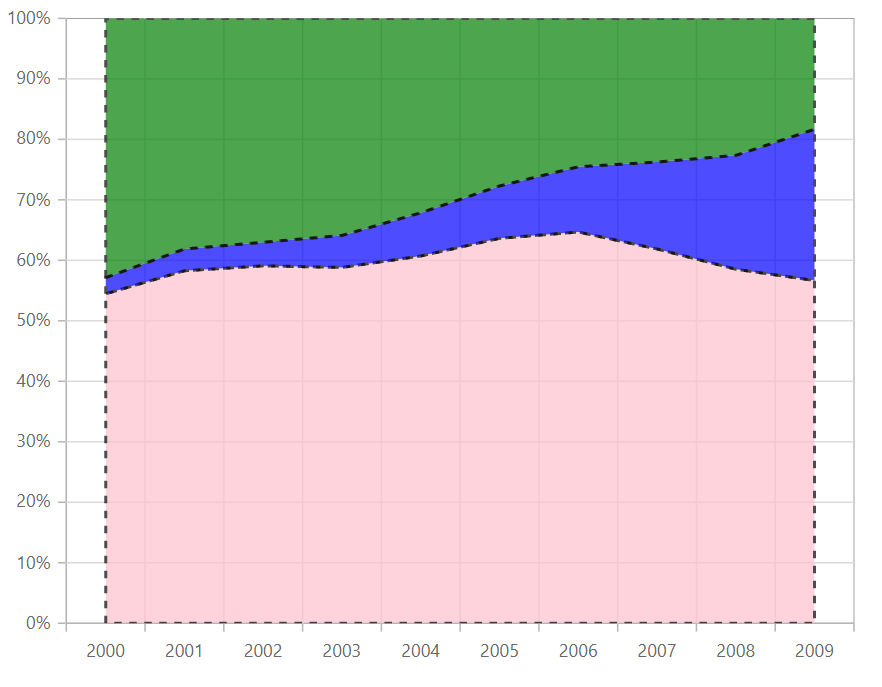# 100% Stacked Area in Blazor Charts Component

11 Aug 20235 minutes to read

## 100% Stacked Area Chart

100% Stacked Area Chart displays multiple series of data as stacked areas, ensuring that the cumulative proportion of each stacked element always totals 100%. Hence, the y-axis will always be rendered with the range 0–100%. To render a 100% Stacked Area series, set the series Type as StackingArea100.

``````@using Syncfusion.Blazor.Charts

<SfChart>
<ChartPrimaryXAxis ValueType="Syncfusion.Blazor.Charts.ValueType.Category"/>

<ChartSeriesCollection>
<ChartSeries DataSource="@MedalDetails" XName="X" YName="Y" Type="ChartSeriesType.StackingArea100"/>
<ChartSeries DataSource="@MedalDetails" XName="X" YName="Y1" Type="ChartSeriesType.StackingArea100"/>
<ChartSeries DataSource="@MedalDetails" XName="X" YName="Y2" Type="ChartSeriesType.StackingArea100"/>
</ChartSeriesCollection>
</SfChart>

@code{
public class ChartData
{
public double X { get; set; }
public double Y { get; set; }
public double Y1 { get; set; }
public double Y2 { get; set; }
}

public List<ChartData> MedalDetails = new List<ChartData>
{
new ChartData{ X=2000, Y= 0.61, Y1= 0.03, Y2= 0.48},
new ChartData{ X=2001, Y= 0.81, Y1= 0.05, Y2= 0.53 },
new ChartData{ X=2002, Y= 0.91, Y1= 0.06, Y2= 0.57 },
new ChartData{ X=2003, Y= 1, Y1= 0.09, Y2= 0.61 },
new ChartData{ X=2004, Y= 1.19, Y1= 0.14, Y2= 0.63 },
new ChartData{ X=2005, Y= 1.47, Y1= 0.20, Y2= 0.64 },
new ChartData{ X=2006, Y= 1.74, Y1= 0.29, Y2= 0.66 },
new ChartData{ X=2007, Y= 1.98, Y1= 0.46, Y2= 0.76 },
new ChartData{ X=2008, Y= 1.99, Y1= 0.64, Y2= 0.77 },
new ChartData{ X=2009, Y= 1.70, Y1= 0.75, Y2= 0.55 }
};
}``````NOTE

Refer to our Blazor 100% Stacked Area Chart feature tour page to know about its other groundbreaking feature representations. Explore our Blazor 100% Stacked Area Chart Example to know how to render and configure the 100% Stacked Area type chart.

## Series customization

The following properties can be used to customize the 100% Stacked Area series.

``````@using Syncfusion.Blazor.Charts

<SfChart>
<ChartPrimaryXAxis ValueType="Syncfusion.Blazor.Charts.ValueType.Category" />

<ChartSeriesCollection>
<ChartSeries DataSource="@MedalDetails" XName="X" YName="Y" Fill="pink" Opacity="0.7" DashArray="5,5" Type="ChartSeriesType.StackingArea100">
<ChartSeriesBorder Width="2" Color="black"></ChartSeriesBorder>
</ChartSeries>
<ChartSeries DataSource="@MedalDetails" XName="X" YName="Y1" Fill="blue" Opacity="0.7" DashArray="5,5" Type="ChartSeriesType.StackingArea100">
<ChartSeriesBorder Width="2" Color="black"></ChartSeriesBorder>
</ChartSeries>
<ChartSeries DataSource="@MedalDetails" XName="X" YName="Y2" Fill="green" Opacity="0.7" DashArray="5,5" Type="ChartSeriesType.StackingArea100">
<ChartSeriesBorder Width="2" Color="black"></ChartSeriesBorder>
</ChartSeries>
</ChartSeriesCollection>
</SfChart>

@code{
public class ChartData
{
public double X { get; set; }
public double Y { get; set; }
public double Y1 { get; set; }
public double Y2 { get; set; }
}

public List<ChartData> MedalDetails = new List<ChartData>
{
new ChartData{ X=2000, Y= 0.61, Y1= 0.03, Y2= 0.48},
new ChartData{ X=2001, Y= 0.81, Y1= 0.05, Y2= 0.53 },
new ChartData{ X=2002, Y= 0.91, Y1= 0.06, Y2= 0.57 },
new ChartData{ X=2003, Y= 1, Y1= 0.09, Y2= 0.61 },
new ChartData{ X=2004, Y= 1.19, Y1= 0.14, Y2= 0.63 },
new ChartData{ X=2005, Y= 1.47, Y1= 0.20, Y2= 0.64 },
new ChartData{ X=2006, Y= 1.74, Y1= 0.29, Y2= 0.66 },
new ChartData{ X=2007, Y= 1.98, Y1= 0.46, Y2= 0.76 },
new ChartData{ X=2008, Y= 1.99, Y1= 0.64, Y2= 0.77 },
new ChartData{ X=2009, Y= 1.70, Y1= 0.75, Y2= 0.55 }
};
}``````NOTE

Refer to our Blazor Charts feature tour page for its groundbreaking feature representations and also explore our Blazor Chart Example to know various chart types and how to represent time-dependent data, showing trends at equal intervals.